# Normal Equation in Linear Regression

## ML | Normal Equation in Linear Regression

Normal Equation is an analytical approach to Linear Regression with a Least Square Cost Function. We can directly find out the value of θ without using Gradient Descent. Following this approach is an effective and time-saving option when are working with a dataset with small features.
Normal Equation is a follows :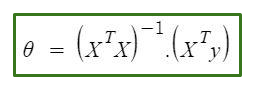In the above equation,
θ: hypothesis parameters that define it the best.
X: Input feature value of each instance.
Y: Output value of each instance.

#### Maths Behind the equation –

Given the hypothesis function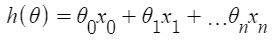where,
n: the no. of features in the data set.
x0: 1 (for vector multiplication)
Notice that this is a dot product between θ and x values. So for the convenience to solve we can write it as :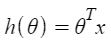The motive in Linear Regression is to minimize the cost function :

J(\Theta) = \frac{1}{2m} \sum_{i = 1}^{m} \frac{1}{2} [h_{\Theta}(x^{(i)}) – y^{(i)}]^{2}

where,
xi: the input value of iih training example.
m: no. of training instances
n: no. of data-set features
yi: the expected result of ith instance
Let us representing the cost function in a vector form.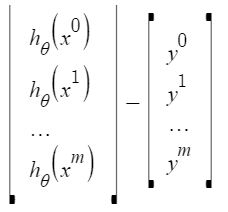we have ignored 1/2m here as it will not make any difference in the working. It was used for mathematical convenience while calculation gradient descent. But it is no more needed here.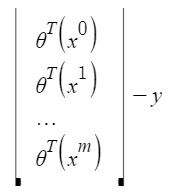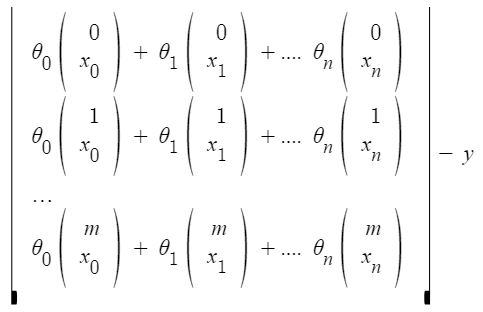xij: value of jih feature in iih training example.
This can further be reduced toBut each residual value is squared. We cannot simply square the above expression. As the square of a vector/matrix is not equal to the square of each of its values. So to get the squared value, multiply the vector/matrix with its transpose. So, the final equation derived is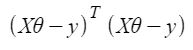Therefore, the cost function is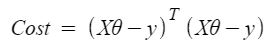So, now getting the value of θ using derivative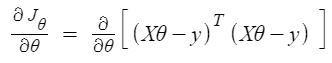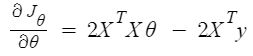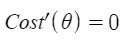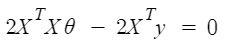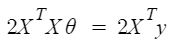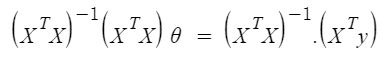So, this is the finally derived Normal Equation with θ giving the minimum cost value.

#### Example:

 # This code may not run on GFG IDE # as required modules not found. # import required modules import numpy as np import matplotlib.pyplot as plt from sklearn.datasets import make_regression # Create data set. x,y=make_regression(n_samples=100,n_features=1,n_informative=1,noise = 10,random_state=10) # Plot the generated data set. plt.scatter(x,y,s=30,marker='o') plt.xlabel("Feature_1 --->") plt.ylabel("Target_Variable --->") plt.title('Simple Linear Regression') plt.show() # Convert  target variable array from 1d to 2d. y=y.reshape(100,1)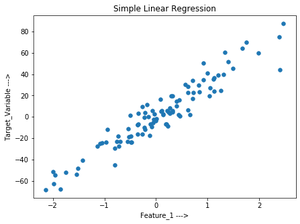#### Let’s implement  the Normal Equation:

 # code # Adding x0=1 to each instance x_new=np.array([np.ones(len(x)),x.flatten()]).T # Using Normal Equation. theta_best_values=np.linalg.inv(x_new.T.dot(x_new)).dot(x_new.T).dot(y) # Display best values obtained. print(theta_best_values)
[[ 0.52804151]
[30.65896337]]

#### Try to predict for new data instance:

 # code # sample data instance. x_sample=np.array([[-2],]) # Adding x0=1 to each instance. x_sample_new=np.array([np.ones(len(x_sample)),x_sample.flatten()]).T # Display the sample. print("Before adding x0:\n",x_sample) print("After adding x0:\n",x_sample_new)
Before adding x0:
[[-2]
[ 4]]
[[ 1. -2.]
[ 1.  4.]]
 # code # predict the values for given data instance. predict_value=x_sample_new.dot(theta_best_values) print(predict_value)
[[-60.78988524]
[123.16389501]]

#### Plot the output:

 # code # Plot the output. plt.scatter(x,y,s=30,marker='o') plt.plot(x_sample,predict_value,c='red') plt.plot() plt.xlabel("Feature_1 --->") plt.ylabel("Target_Variable --->") plt.title('Simple Linear Regression') plt.show()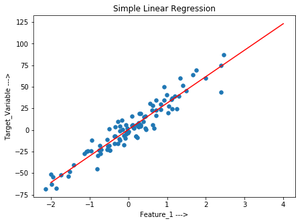#### Verify the above using sklearn LinearRegression class:

 # code # Verification. from sklearn.linear_model import LinearRegression lr=LinearRegression()    # Object. lr.fit(x,y)              # fit method. # Print obtained theta values. print("Best value of theta:",lr.intercept_,lr.coef_,sep='\n') #predict. print("predicted value:",lr.predict(x_sample),sep='\n')
Best value of theta:
[0.52804151]
[[30.65896337]]

predicted value:
[[-60.78988524]
[123.16389501]]

Last Updated on March 1, 2022 by admin

## Python – Convert list into list of listsPython – Convert list into list of lists

Python | Convert list into list of lists Given a list of strings, write a

## Classifying data using Support Vector Machines(SVMs) in PythonClassifying data using Support Vector Machines(SVMs) in Python

Classifying data using Support Vector Machines(SVMs) in Python Introduction to SVMs: In machine learning, support

## sys.stdout.write in Pythonsys.stdout.write in Python

sys.stdout.write in Python This is a built-in Python module that contains parameters specific to the

## Get minimum values in rows or columns with their index position in Pandas-DataframeGet minimum values in rows or columns with their index position in Pandas-Dataframe

Get minimum values in rows or columns with their index position in Pandas-Dataframe Let’s discuss

## Get directory of current Python scriptGet directory of current Python script

Get directory of current Python script While working with file handling you might have noticed that files

## Pretty Print JSON in PythonPretty Print JSON in Python

Pretty Print JSON in Python JSON is a javascript notation of storing and fetching the data.

## exec() in Pythonexec() in Python

exec() in Python exec() function is used for the dynamic execution of Python program which can

## Deploy Python Flask App on HerokuDeploy Python Flask App on Heroku

Deploy Python Flask App on Heroku Flask is a web application framework written in Python.